# Online Civil Engineering Test - Previous UPSC Exam Papers Test 2Loading Test...

Instruction:

• This is a FREE online test. DO NOT pay money to anyone to attend this test.
• Total number of questions : 20.
• Time alloted : 30 minutes.
• Each question carry 1 mark, no negative marks.
• DO NOT refresh the page.
• All the best :-).

1.

To generate 10, 000 hp under a head of 81 m while working at a speed of 500 rpirt, the turbine of choice would be

A.
 PeltonB.
 KaplanC.
 BulbD.
 Francis2.

The given figure shows gradually varied flow in an open channel with a beak in bed slope. Types of water surface profiles occurring from left to right are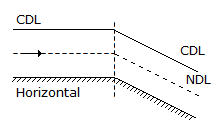A.
 H2 , S3B.
 H2 , S2C.
 H2 , M2D.
 H3 , M23.

Consider the following methods of preservation of timber :
1. Dipping
2. Brushing or spraying
3. Pressure impregnation
The correct sequence in decreasing order of the effectiveness of these methods of preservation is

A.
 1, 2, 3B.
 2, 1, 3C.
 3, 1, 2D.
 3, 2, 14.

While driving a large number of piles in loose sand :

A.
 It is advantageous to follow a sequence of pile driving such that the inner piles are driven first and then proceed outwardsB.
 It is advantageous to follow a sequence of pile driving such that the piles near the periphery are driven first and inner piles are driven laterC.
 It is advantageous to follow a sequence of pile driving such that alternately inner and outer piles are drivenD.
 Driving of piles can be done in any random order5.

Consider the following statement: High early strength of cement is obtained as a result of
1. fine grinding.
2. decreasing the lime content
3. burning at higher temperatures
4. increasing the quantity of gypsum.
Of these statements :

A.
 1 and 2 are correctB.
 1 and 3 are correctC.
 2, 3 and 4 are correctD.
 1, 3 and 4 are correct6.

The moment of inertia of a given rectangular area is minimum about

A.
 its longer centroidal axisB.
 its polar axisC.
 its axis along the diagonalD.
 its shorter centroidal axis7.

Which one of the following statements is correct ? Compared to IFR conditions, the runaway capacity of a runway operating under VFR consitions

A.
 is higherB.
 is lowerC.
 is the sameD.
 cannot be generalised8.

An open cylindrical vessel with axis vertical and filled with a liquid is falling freely with an acceleration What will be the absolute pressure at the base of the vessel ?

A.
 Equal to liquid columnB.
 Below atmospheric pressureC.
 Above atmosphere pressureD.
 Equal to atmosphere pressure9.

A rigid bar is supported by a spring as shown in the given figure.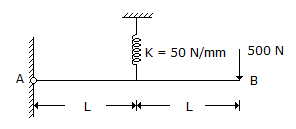The deflection of the point B will be

A.
 10 mm upwardB.
 20 mm downwardC.
 5 mm upwardD.
 40 mm downward.Explanation:

The Force in spring F = (500x2L)/L = 1000N.

Deflection in the spring δ = F/k = 1000/50 = 20mm.

Deflection at the point B = 2δ = 40mm downward.

10.

In a direct shear test the shear stress and normal stress on a dry sand sample at failure are 0.6 kg/cm2 and 1 kg/cm2 respectively. The angle of internal friction of the sand will be nearly

A.
 25°B.
 31°C.
 37°D.
 43°11.

A short column of external diameter D1 and internal diameter D2 carries an external load W. For no-tension condition, the eccentricity will be :

A.
 (D12 - D22)/8D2B.
 (D21 - D22)/8D2C.
 (D12 - D22)/8D1D.
 (D1 - D2)2 /8D112.

For fish habitat in a river, the minimum dissolved oxygen required is

A.
 2 mg/lB.
 4 mg/lC.
 8 mg/lD.
 10 mgl13.

The ratio of the torsional moments of resistance of a solid circular shaft of diameter D, and a hollow circular shaft having external diameter D and internal diameter d is given by

A.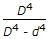B.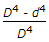C.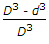D.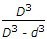14.

In the schematic flownet shown in the given figure the hydraulic potential at point A is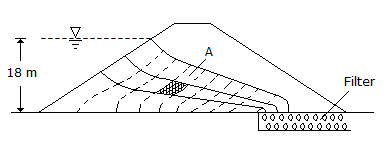A.
 5 m of waterB.
 12 m of waterC.
 15 m of waterD.
 25 m of water15.

Following data refer to precise measurement of an angle A on the field :
(i) 2A = 20°10' - weight 2
(ii) 4A = 40°10' - weight 3
What is the most probable value of angle A ?

A.
 10°01'06"B.
 10°02'07"C.
 10°02'51"D.
 10°22'51"16.

Which one of the following is the reaction of the cantilever at B as shown in the figure ?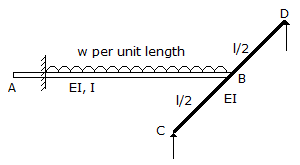A.
 3/8 wlB.
 5/8 wlC.
 6/17 wlD.
 3/21 wl17.

The upper limit of area ratio for which the amount of disturbance of soil sample can be considered to be small is

A.
 10%B.
 15%C.
 20%D.
 25%18.

Consider the following statements :
1. In a member subjected to uniaxial tensile force the maximum normal stress is the external load divided by the maximum cross-sectional area.
2. When the structural member is subjected to uniaxial loading, the shear stress is zero on a plane where the normal stress is maximum.
3. In a member subjected to uniaxial loading, the normal stress on the planes of maximum shear stress is less than the maximum.
Of these statements :

A.
 1 and 2 are correctB.
 1 and 3 are correctC.
 2 and 3 are correctD.
 1, 2 and 3 are correct19.

What is the wave velocity for a uniform train of wave beyond the storm centre for a wave length of 20 m in 14 m deep water ?

A.
 5.5 m/sB.
 11.2 m/sC.
 4.5 m/sD.
 9 m/s20.

The moisture content of a clayey soil is gradually decreased from a large value. What will be the correct sequence of the occurrence of the following limits ?
1. Shrinkage limit
2. Plastic limit
3. Liquid limit
Select the correct answer from the codes given below :

A.
 1, 2, 3B.
 1, 3, 2C.
 3, 2, 1D.
 3, 1, 2